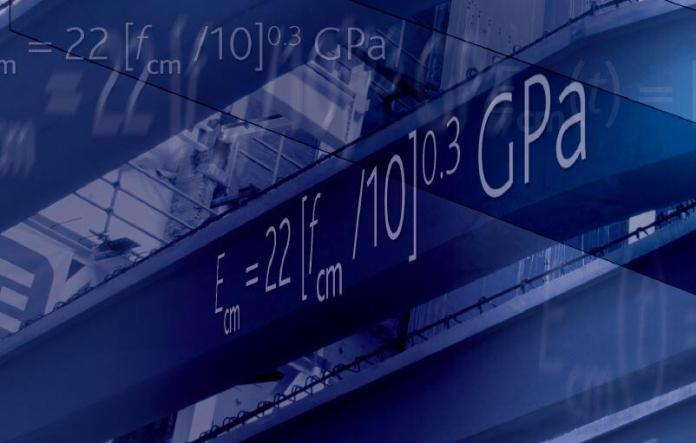# Formulas for Calculating Different Properties of ConcreteThe most basic test done on concrete is the compressive strength test. Sometimes, other properties of concrete such as tensile strength, modulus of elasticity, shrinkage values, etc are needed for design purposes.

Researchers and standards have come up with different relationships between the compressive strength of concrete and other properties. In this article, we are going to show the formulas which relate the compressive strength of concrete with other properties as applicable to the Eurocodes.

## Characteristic strength of concrete (fck)

The characteristic strength is that strength below which 5% of results may be expected to fall during compressive strength test. Individual results below fck may be obtained but, in general, only need to be investigated if they fall more than 4 MPa below fck (BS EN 206-1, cl 8.2, table 14).

## Design strength (fcd)

The compressive design strength of concrete is given by;

fcd = αcc fckc   ——–  (1)

where;
fck = characteristic cylinder compressive strength of concrete at 28 days
γc = partial (safety) factor for concrete (taken as 1.5 for UK National Annex)
αcc = a coefficient taking account of long-term effects on the compressive strength (which is reduced under sustained load) and unfavourable effects resulting from the way the load is applied (conservatively taken as 0.85).

## Target mean strength (fcm)

The target mean strength, fcm, is also the value used to establish the mix design and is intended to take account of the normal variability that will occur in concrete production. This margin of 8MPa for cylinders is consistent with a normal distribution with a standard deviation (SD) of about 5MPa:

fck = fcm – 1.64SD ——- (2)

Where 1.64SD = 8
Therefore SD = 8/1.64 ≈ 5MPa

N/B: For cubes, the margin is 10 MPa which gives a standard deviation of about of 6 MPa.

## Development of compressive strength with time

While design is usually based on the 28-day strength, BS EN 1992-1-1, sub-clause 3.1.2(6) gives an expression for the development of the mean compressive strength of concrete with time at 20°C as follows:

fcm(t) = [βcc(t)]fcm  ——— (3)

where;
fcm(t) is the mean compressive strength at age t days.
βcc(t) = exp{s[1 – (28/t)0.5]} ——— (3a)

where;
s is a coefficient which depends on cement type
= 0.20 for cement of strength classes CEM 42.5R, CEM 52.5N and CEM 52.5R (Class R)
= 0.25 for cement of strength classes CEM 32.5R, CEM 42.5N (Class N)
= 0.38 for cement of strength classes CEM 32.5N (Class S)
(where Class R = high early strength; Class N = normal early strength; Class S = slow early strength).

## Tensile strength

Tensile strength is commonly defined in one of three ways: direct tensile strength, tensile splitting strength, or flexural strength. For normal structural uses, the mean tensile strength, fctm, is related to the cylinder strength by the expressions:

Strength classes ≤ C50/60
fctm = 0.30 fck(2/3) MPa  ——— (4)

Strength classes > C50/60
fctm = 2.12loge [1 + (fcm)/10] MPa ——— (5)

## Flexural tensile strength

Flexural tensile strength can also be calculated from the mean tensile strength by the following expressions.

The flexural strength is the higher of:

fctm,fl = (1.6 – h/1000)fctm ——— (6)
or,
fctm,fl = fctm

where;
h is the total member depth in mm

## Strength development of tensile strength

BS EN 1992-1-1 provides expressions for calculating tensile strength at different maturities:

fctm(t) = [βcc(t)]α fctm ——— (7)

where:
βcc(t) is as defined in Equation (3a)
α = 1 for t < 28 days
α = 2/3 for t ≥ 28 day

## Modulus of elasticity

In design, the secant modulus, Ecm (in GPa), is derived from the mean compressive strength, fcm (in MPa), from the expression:

Ecm = 22 [fcm /10]0.3 GPa ——— (8)

## Variation of Modulus of Elasticity with age

The variation of modulus of elasticity with time is estimated using the expression:
Ecm(t) = [fcm(t)/fcm]0.3 Ecm ——— (9)

This formulas and relationships in this post are culled from:
Bamforth P., Chisholm D., Gibbs J., Harrison T. (2008): Properties of concrete for use in Eurocode 2. The Concrete Centre, UK